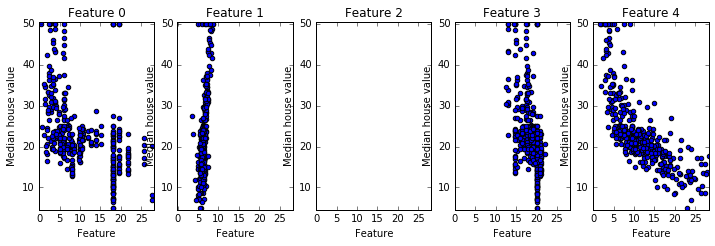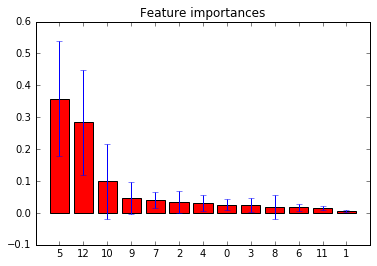## Regression in scikit-learn

We will compare several regression methods by using the same dataset. We will try to predict the price of a house as a function of its attributes.

In :
```import numpy as np
import matplotlib.pyplot as plt
%pylab inline
```
Populating the interactive namespace from numpy and matplotlib

## Import the Boston House Pricing Dataset

In :
```from sklearn.datasets import load_boston
print (boston.data.shape)
print (boston.feature_names)
print (np.max(boston.target), np.min(boston.target), np.mean(boston.target))
```
```(506, 13)
['CRIM' 'ZN' 'INDUS' 'CHAS' 'NOX' 'RM' 'AGE' 'DIS' 'RAD' 'TAX' 'PTRATIO'
'B' 'LSTAT']
50.0 5.0 22.5328063241
```
In :
```print (boston.data)
print (np.max(boston.data), np.min(boston.data), np.mean(boston.data))
```
```[  6.32000000e-03   1.80000000e+01   2.31000000e+00   0.00000000e+00
5.38000000e-01   6.57500000e+00   6.52000000e+01   4.09000000e+00
1.00000000e+00   2.96000000e+02   1.53000000e+01   3.96900000e+02
4.98000000e+00]
711.0 0.0 70.0724468258
```

You should try printing boston.DESCR to get a feel of what each feature means. This is a very healthy habit: machine learning is not just number crunching, understanding the problem we are facing is crucial, especially to select the best learning model to use.

In :
```print (boston.DESCR)
```
```Boston House Prices dataset

Notes
------
Data Set Characteristics:

:Number of Instances: 506

:Number of Attributes: 13 numeric/categorical predictive

:Median Value (attribute 14) is usually the target

:Attribute Information (in order):
- CRIM     per capita crime rate by town
- ZN       proportion of residential land zoned for lots over 25,000 sq.ft.
- INDUS    proportion of non-retail business acres per town
- CHAS     Charles River dummy variable (= 1 if tract bounds river; 0 otherwise)
- NOX      nitric oxides concentration (parts per 10 million)
- RM       average number of rooms per dwelling
- AGE      proportion of owner-occupied units built prior to 1940
- DIS      weighted distances to five Boston employment centres
- TAX      full-value property-tax rate per \$10,000
- PTRATIO  pupil-teacher ratio by town
- B        1000(Bk - 0.63)^2 where Bk is the proportion of blacks by town
- LSTAT    % lower status of the population
- MEDV     Median value of owner-occupied homes in \$1000's

:Missing Attribute Values: None

:Creator: Harrison, D. and Rubinfeld, D.L.

This is a copy of UCI ML housing dataset.
http://archive.ics.uci.edu/ml/datasets/Housing

This dataset was taken from the StatLib library which is maintained at Carnegie Mellon University.

The Boston house-price data of Harrison, D. and Rubinfeld, D.L. 'Hedonic
prices and the demand for clean air', J. Environ. Economics & Management,
vol.5, 81-102, 1978.   Used in Belsley, Kuh & Welsch, 'Regression diagnostics
...', Wiley, 1980.   N.B. Various transformations are used in the table on
pages 244-261 of the latter.

The Boston house-price data has been used in many machine learning papers that address regression
problems.

**References**

- Belsley, Kuh & Welsch, 'Regression diagnostics: Identifying Influential Data and Sources of Collinearity', Wiley, 1980. 244-261.
- Quinlan,R. (1993). Combining Instance-Based and Model-Based Learning. In Proceedings on the Tenth International Conference of Machine Learning, 236-243, University of Massachusetts, Amherst. Morgan Kaufmann.
- many more! (see http://archive.ics.uci.edu/ml/datasets/Housing)

```
###### Separate train and test
In :
```from sklearn.cross_validation import train_test_split
X_train, X_test, y_train, y_test = train_test_split(boston.data, boston.target, test_size=0.25, random_state=33)
```
###### Find the most important features
In :
```from sklearn.feature_selection import *
fs=SelectKBest(score_func=f_regression,k=5)
X_new=fs.fit_transform(X_train,y_train)
print (zip(fs.get_support(),boston.feature_names))

x_min, x_max = X_new[:,0].min() - .5, X_new[:, 0].max() + .5
y_min, y_max = y_train.min() - .5, y_train.max() + .5
#fig=plt.figure()
#fig.subplots_adjust(left=0, right=1, bottom=0, top=1, hspace=0.05, wspace=0.05)

# Two subplots, unpack the axes array immediately
fig, axes = plt.subplots(1,5)
fig.set_size_inches(12,12)

for i in range(5):
axes[i].set_aspect('equal')
axes[i].set_title('Feature ' + str(i))
axes[i].set_xlabel('Feature')
axes[i].set_ylabel('Median house value')
axes[i].set_xlim(x_min, x_max)
axes[i].set_ylim(y_min, y_max)
sca(axes[i])
plt.scatter(X_new[:,i],y_train)
```
```<zip object at 0x000002557E183788>
```### Normalize data

In :
```from sklearn.preprocessing import StandardScaler
scalerX = StandardScaler().fit(X_train)
scalery = StandardScaler().fit(y_train)

X_train = scalerX.transform(X_train)
y_train = scalery.transform(y_train)
X_test = scalerX.transform(X_test)
y_test = scalery.transform(y_test)

print (np.max(X_train), np.min(X_train), np.mean(X_train), np.max(y_train), np.min(y_train), np.mean(y_train))
```
```10.2028980046 -4.66702040845 2.47038706385e-15 2.91774920367 -1.93147098641 3.58552238032e-16
```
```C:\Anaconda3\lib\site-packages\sklearn\preprocessing\data.py:583: DeprecationWarning: Passing 1d arrays as data is deprecated in 0.17 and will raise ValueError in 0.19. Reshape your data either using X.reshape(-1, 1) if your data has a single feature or X.reshape(1, -1) if it contains a single sample.
warnings.warn(DEPRECATION_MSG_1D, DeprecationWarning)
C:\Anaconda3\lib\site-packages\sklearn\preprocessing\data.py:646: DeprecationWarning: Passing 1d arrays as data is deprecated in 0.17 and will raise ValueError in 0.19. Reshape your data either using X.reshape(-1, 1) if your data has a single feature or X.reshape(1, -1) if it contains a single sample.
warnings.warn(DEPRECATION_MSG_1D, DeprecationWarning)
C:\Anaconda3\lib\site-packages\sklearn\preprocessing\data.py:646: DeprecationWarning: Passing 1d arrays as data is deprecated in 0.17 and will raise ValueError in 0.19. Reshape your data either using X.reshape(-1, 1) if your data has a single feature or X.reshape(1, -1) if it contains a single sample.
warnings.warn(DEPRECATION_MSG_1D, DeprecationWarning)
```

Accuracy is not a good idea in regression as metrics, since we are predicting real values, it is almost impossible for us to predict exactly the final value.

There are several measures that can be used (you can look at the list of functions under sklearn.metrics module). The most common is the R2 score, or coefficient of determination that measures the proportion of the outcomes variation explained by the model, and is the default score function for regression methods in scikit-learn.

This score reaches its maximum value of 1 when the model perfectly predicts all the test target values.

Using this measure, we will build a function that trains a model and evaluates its performance using five-fold cross-validation and the coefficient of determination

In :
```from sklearn.cross_validation import *
def train_and_evaluate(clf, X_train, y_train):

clf.fit(X_train, y_train)

print ("Coefficient of determination on training set:",clf.score(X_train, y_train))

# create a k-fold croos validation iterator of k=5 folds
cv = KFold(X_train.shape, 5, shuffle=True, random_state=33)
scores = cross_val_score(clf, X_train, y_train, cv=cv)
print ("Average coefficient of determination using 5-fold crossvalidation:",np.mean(scores))
```

### a linear model

The question that linear models try to answer is which hyperplane in the 14-dimensional space created by our learning features (including the target value) is located closer to them. After this hyperplane is found, prediction reduces to calculate the projection on the hyperplane of the new point, and returning the target value coordinate.

The usual measure is least squares: calculate the distance of each instance to the hyperplane, square it (to avoid sign problems), and sum them. The hyperplane whose sum is smaller is the least squares estimator (the hyperplane in the case if two dimensions are just a line).

Since we don’t know how our data fits (it is difficult to print a 14-dimension scatter plot!), we will start with a linear model called SGDRegressor. SGDRegressor tries to find the hyperplane that minimizes a certain loss function (typically, the sum of squared distances from each instance to the hyperplane). It uses Stochastic Gradient Descent to find the minimum.

In :
```from sklearn import linear_model
clf_sgd = linear_model.SGDRegressor(loss='squared_loss', penalty=None,  random_state=42)
train_and_evaluate(clf_sgd,X_train,y_train)
```
```Coefficient of determination on training set: 0.743617732983
Average coefficient of determination using 5-fold crossvalidation: 0.710809853468
```

We can print the hyperplane coefficients our method has calculated, which is as follows

In :
```print (clf_sgd.coef_)
```
```[-0.08527595  0.06706144 -0.05032898  0.10874804 -0.07755151  0.38961893
-0.02485839 -0.20990016  0.08491659 -0.05495108 -0.19854006  0.06126093
-0.37817963]
```

You probably noted the penalty=None parameter when we called the method. The penalization parameter for linear regression methods is introduced to avoid overfitting. It does this by penalizing those hyperplanes having some of their coefficients too large, seeking hyperplanes where each feature contributes more or less the same to the predicted value. This parameter is generally the L2 norm (the squared sums of the coefficients) or the L1 norm (that is the sum of the absolute value of the coefficients). Let’s see how our model works if we introduce an L2 penalty.

In :
```clf_sgd1 = linear_model.SGDRegressor(loss='squared_loss', penalty='l2',  random_state=42)
train_and_evaluate(clf_sgd1,X_train,y_train)
```
```Coefficient of determination on training set: 0.743616743208
Average coefficient of determination using 5-fold crossvalidation: 0.71081206667
```
In :
```clf_sgd2 = linear_model.SGDRegressor(loss='squared_loss', penalty='l1',  random_state=42)
train_and_evaluate(clf_sgd2,X_train,y_train)
```
```Coefficient of determination on training set: 0.74358692291
Average coefficient of determination using 5-fold crossvalidation: 0.710763609874
```
In :
```clf_sgd3 = linear_model.SGDRegressor(loss='squared_loss', penalty='elasticnet',  random_state=42)
train_and_evaluate(clf_sgd3,X_train,y_train)
```
```Coefficient of determination on training set: 0.743612281878
Average coefficient of determination using 5-fold crossvalidation: 0.710804829162
```

#### Ridge regression

In :
```clf_ridge = linear_model.Ridge()
train_and_evaluate(clf_ridge,X_train,y_train)
```
```Coefficient of determination on training set: 0.754904554125
Average coefficient of determination using 5-fold crossvalidation: 0.714014174328
```

In this case, we did not obtain an improvement

#### Support Vector Machines for regression

The regression version of SVM can be used instead to find the hyperplane.

In :
```from sklearn import svm
clf_svr= svm.SVR(kernel='linear')
train_and_evaluate(clf_svr,X_train,y_train)
```
```Coefficient of determination on training set: 0.71886923342
Average coefficient of determination using 5-fold crossvalidation: 0.707838419194
```

Here, we had no improvement. However, one of the main advantages of SVM is that (using what we called the kernel trick) we can use a nonlinear function, for example, a polynomial function to approximate our data.

In :
```clf_svr_poly= svm.SVR(kernel='poly')
train_and_evaluate(clf_svr_poly,X_train,y_train)
```
```Coefficient of determination on training set: 0.904109273301
Average coefficient of determination using 5-fold crossvalidation: 0.779288545488
```

Now, our results are six points better in terms of coefficient of determination. We can actually improve this by using a Radial Basis Function (RBF) kernel.

In :
```clf_svr_rbf= svm.SVR(kernel='rbf')
train_and_evaluate(clf_svr_rbf,X_train,y_train)
```
```Coefficient of determination on training set: 0.900132065979
Average coefficient of determination using 5-fold crossvalidation: 0.833662221567
```

RBF kernels have been used in several problems and have shown to be very effective. Actually, RBF is the default kernel used by SVM methods in scikit-learn.

### Random Forests

When used for regression, the tree growing procedure is exactly the same, but at prediction time, when we arrive at a leaf, instead of reporting the majority class, we return a representative real value, for example, the average of the target values.

Actually, we will use Extra Trees, implemented in the ExtraTreesRegressor class within the sklearn.ensemble module. This method adds an extra level of randomization. It not only selects for each tree a different, random subset of features, but also randomly selects the threshold for each decision.

In :
```from sklearn import ensemble
clf_et=ensemble.ExtraTreesRegressor(n_estimators=10,random_state=42)
train_and_evaluate(clf_et,X_train,y_train)
```
```Coefficient of determination on training set: 1.0
Average coefficient of determination using 5-fold crossvalidation: 0.861758978344
```

The first thing to note is that we have not only completely eliminated underfitting (achieving perfect prediction on training values), but also improved the performance by three points while using cross-validation. An interesting feature of Extra Trees is that they allow computing the importance of each feature for the regression task.

In :
```importances = clf_et.feature_importances_
std = np.std([tree.feature_importances_ for tree in clf_et.estimators_],
axis=0)
indices = np.argsort(importances)[::-1]

# Print the feature ranking
print("Feature ranking:")

for f in range(X_train.shape):
print("%d. feature %d (%f)" % (f + 1, indices[f], importances[indices[f]]))

# Plot the feature importances of the forest
plt.figure()
plt.title("Feature importances")
plt.bar(range(X_train.shape), importances[indices],
color="r", yerr=std[indices], align="center")
plt.xticks(range(X_train.shape), indices)
plt.xlim([-1, X_train.shape])
plt.show()
```
```Feature ranking:
1. feature 5 (0.358145)
2. feature 12 (0.284215)
3. feature 10 (0.099512)
4. feature 9 (0.046619)
5. feature 7 (0.039713)
6. feature 2 (0.034406)
7. feature 4 (0.031874)
8. feature 0 (0.025733)
9. feature 3 (0.023603)
10. feature 8 (0.018942)
11. feature 6 (0.017053)
12. feature 11 (0.015143)
13. feature 1 (0.005044)
```In :
```print (list(zip(clf_et.feature_importances_,boston.feature_names)))
```
```[(0.025733049004581798, 'CRIM'), (0.0050438532027558842, 'ZN'), (0.034405644939308928, 'INDUS'), (0.023602561777571307, 'CHAS'), (0.031874162235100457, 'NOX'), (0.35814513144036819, 'RM'), (0.017052578400506287, 'AGE'), (0.039713133345196064, 'DIS'), (0.018941821085751577, 'RAD'), (0.046618521397262996, 'TAX'), (0.099511801492762245, 'PTRATIO'), (0.015142513715149682, 'B'), (0.28421522796368465, 'LSTAT')]
```

### Evaluation

In :
```from sklearn import metrics
def measure_performance(X,y,clf, show_accuracy=True, show_classification_report=True, show_confusion_matrix=True, show_r2_score=False):
y_pred=clf.predict(X)
if show_accuracy:
print ("Accuracy:{0:.3f}".format(metrics.accuracy_score(y,y_pred)),"\n")

if show_classification_report:
print ("Classification report")
print (metrics.classification_report(y,y_pred),"\n")

if show_confusion_matrix:
print ("Confusion matrix")
print (metrics.confusion_matrix(y,y_pred),"\n")

if show_r2_score:
print ("Coefficient of determination:{0:.3f}".format(metrics.r2_score(y,y_pred)),"\n")

measure_performance(X_test,y_test,clf_et, show_accuracy=False, show_classification_report=False,show_confusion_matrix=False, show_r2_score=True)
```
```Coefficient of determination:0.802

excerpt from
```

This site uses Akismet to reduce spam. Learn how your comment data is processed.